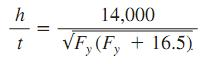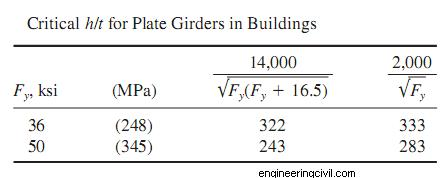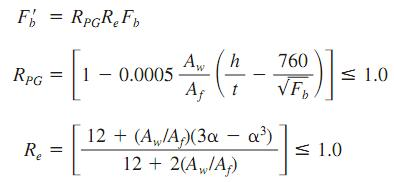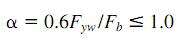Awarded as the best online publication by CIDC

# Plate Girder In Buildings

For greatest resistance to bending, as much of a plate girder cross section as practicable should be concentrated in the flanges, at the greatest distance from the neutral axis. This might require, however, a web so thin that the girder would fail by web buckling before it reached its bending capacity.

To preclude this, the AISC specification limits h/t.

For an unstiffened web, this ratio should not exceed.where Fy = yield strength of compression flange,ksi (MPa).

Larger values of h/t may be used, however, if the web is stiffened at appropriate intervals.

For this purpose, vertical angles may be fastened to the web or vertical plates welded to it. These transverse stiffeners are not required, though, when h/t is less than the value computed from the preceding equation or following tableWith transverse stiffeners spaced not more than 1.5 times the girder depth apart, the web clear-depth/thickness ratio may be as large as
h/t=2000/(Fy)½

If, however, the web depth/thickness ratio h/t exceeds 760/(Fb)½, where Fb, ksi (MPa), is the allowable bending stress in the compression flange that would ordinarily apply, this stress should be reduced to F b , given by the following equations:Where Aw= web area , in2(mm2 )
Af= area of compression flange,in2(mm2)
Fyw =minimum specified yield stress, ksi, (MPa), of web steelIn a hybrid girder, where the flange steel has a higher yield strength than the web, the preceding equation protects against excessive yielding of the lower strength web in the vicinity of the higher strength flanges. For nonhybrid girders, Re= 1.0.